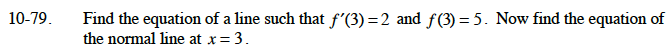### Home > CALC > Chapter 10 > Lesson 10.1.8 > Problem10-79

10-79.

Find the equation of a line such that f ′(3) = 2 and f(3) = 5. Now find the equation of the normal line at x = 3. Homework Help ✎This is a line with a slope of 2 passing through the point (3, 5).

"Normal" means perpendicular. The slope of the normal line is –1/2.